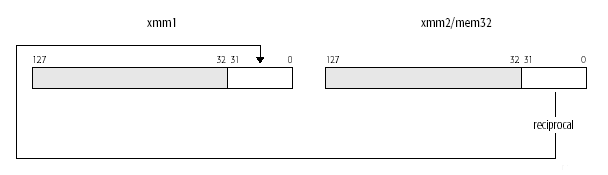A basic building block operation in geometry involves computing divisions and square roots. For instance, transformation often involves dividing each x, y, z coordinate by the W perspective coordinate; normalization is another common geometry operation, which requires the computation of 1/square-root. In order to optimize these cases, SSE introduces two approximation instructions: RCP and RSQRT. These instructions are implemented via hardware lookup tables and are inherently less precise (12 bits of mantissa) than the full IEEE-compliant DIV and SQRT (24 bits of mantissa), but have the advantage of being much faster than the full precision versions. When greater precision is needed, the approximation instructions can be used with a single Newton-Raphson iteration to achieve almost the same precision as the IEEE instructions (~22 bits of mantissa). For a basic geometry pipeline, these instructions can improve overall performance on the order of 15%.